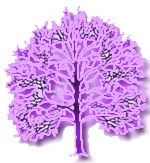cross-referenced news and research resources about

# mathematical theories and theoretical mathematics### schema-root.org

theories

maths:
theories

cross-references for
theories:

mathematical theories